• Call Now

1800-102-2727•

# Vernier Caliper - Measurement, Least Count, Zero Error

Is it possible to measure lengths smaller than millimeter by a scale whose smallest calibration is millimeter? You might think no, but we can indeed do this by making a few arrangements! Let’s see how in this article. For this the widely used instruments are vernier caliper and screw gauge. They also involve some degree of error. These errors come due to improper usage or wear and tear. While analysing the error and correcting it we can have near perfect results.

## Vernier Caliper

To measure length up to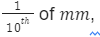we can use Vernier caliper. It consists of three parts.Main scale (M):

It is a steel metallic strip, graduated in Cm and mm  at one edge. It carries two fixed jaws M1 and M2

Vernier scale (V):

V is the vernier scale which can slide over the main scale M. Vernier scale can be fixed at any position with the help of screw S. The vernier scale has 10 divisions over the length of 9 mm on the main scale. Vernier scale has two jaws v1 and v2. When pushed towards M1 and M2, V1 touches M1  and v2 touches M2. In this position, the instrument should be free from any error. The zeros of the vernier scale and the main scale should coincide. For external measurements the jaws M1 and V1 is used and for internal measurements, jaws M2 and v2 is used.

Metallic Strip D:

There is a thin metallic strip D attached to the backside of the main scale M. It is connected with the vernier scale V. When the jaws of main scale and the vernier scale touch each other, the edge of D touches the edge of main scale M When the jaws are separated, D moves out. This metallic strip D is used to measure depth.

Now, suppose N Vernier Scale Divisions coincide with m Main Scale Divisions. Then, the same can be written as,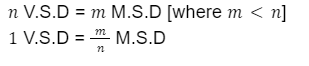The difference between 1 MSD and 1 VSD is called the vernier constant (VC) or the least count (LC). This is the smallest distance that can be accurately measured with the help of vernier scale.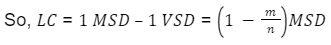## Measuring with a Vernier Caliper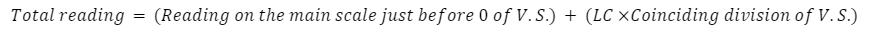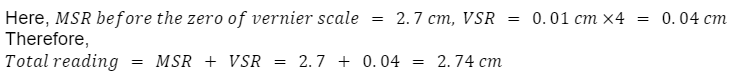While measuring with the vernier caliper we can also stumble upon zero error.

## Zero Error in Vernier Caliper

If the zero marks in the main scale and in the vernier scale don’t coincide at the time when V1 and V2touch each other, it has zero error. Zero error is subtracted from the total reading to get correct resultsIn positive zero error(+Z), the vernier zero mark will lie right to the main scale zero mark when the jaws touch each other.

In negative zero error(-Z), the vernier zero mark will lie left to the main scale zero mark when the jaws touch each other.

Zero error is also counted as any reading in the vernier caliper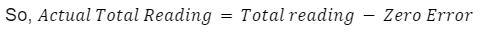## Practice Problems of Vernier Caliper

Question 1. In a vernier caliper, when the jaws are touching each other the zero marks in both the scale coincides, 10 vernier scale divisions matches with 9 main scale divisions (in mm). A rod of length L is measured with this vernier caliper. It shows 55 in main scale reading and in the vernier scale 8th mark matches with a main scale division. What is the value of L?

Answer: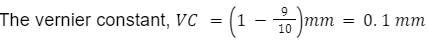Here the zero mark in vernier scale matches with the zero mark in main scale. So, no zero error exists.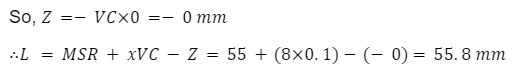Question 2. In a vernier caliper, when the jaws are touching each other the zero mark on the vernier scale matches with the zero mark on the main scale division. 10 vernier scale divisions matches with 9 main scale divisions (in MM). A rod of length L is measured with this vernier caliper. It shows 58 in main scale reading and in the vernier scale 8th mark matches with a main scale division. What is the value of L?

Answer: The vernier constant,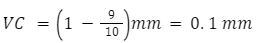Here the zero mark in vernier scale matches with the zero mark in main scale. So, no zero error exists.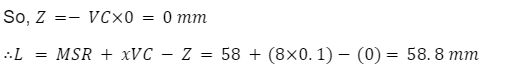Question 3. In a vernier caliper, when the jaws are touching each other the zero mark on the vernier scale is left behind slightly of the zero mark on the main scale. At that moment the 5th division mark on vernier caliper matches perfectly with a division on the main scale. When the zero marks in both the scale coincides, 10 vernier scale divisions matches with 9 main scale divisions (in mm). A rod of length l is measured with this vernier caliper. It shows 55 in main scale reading and in the vernier scale 8th mark matches with a main scale division. What is the value of L?

Answer: The vernier constant,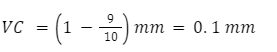Here the zero mark in vernier scale is left behind the zero mark in main scale. So, a negative zero error exists.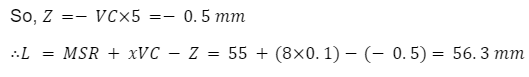Question 4. In a vernier caliper, when the jaws are touching each other the zero mark on the vernier scale goes beyond slightly of the zero mark of the main scale. At that moment the 6h division mark on vernier caliper matches perfectly with a main scale division. When the zero marks in both the scale coincides, 10 vernier scale divisions matches with 9 main scale divisions (in mm). A rod of length L is measured with this vernier caliper. It shows 58 in main scale reading and in the vernier scale 8th mark matches with a main scale division. What is the value of L?

Answer: The vernier constant,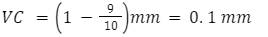Here the zero mark on the vernier scale goes beyond the zero mark on the main scale. So, a positive zero error exists.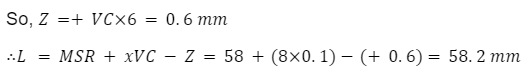## FAQs of Vernier Caliper

Question 1. What is meant by the least count?

Answer: The difference between 1 MSD and 1VSD is called the vernier constant (VC) or the least count (LC). This is the smallest distance that can be accurately measured with the help of vernier scale.

Question 2. What are the lower jaws of a vernier caliper used for?

Answer: The lower jaws are used to measure the outer diameter of objects like a sphere or a cylinder.

Question 3. The vernier caliper is used to measure length with accuracy upto the

a. screw pitch

b. vernier constant

Question 4. In a vernier caliper the main scale is of mm range and 20  vernier divisions match with the 19 main scale divisions. The least count is

a. 0.5 mm

b. 0.03

c. 0.2 mm

d. 0.05 mm